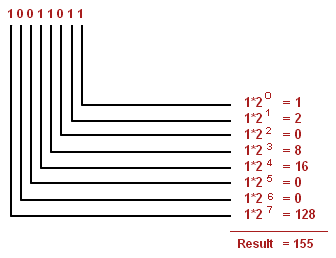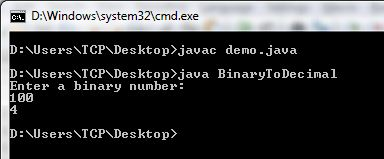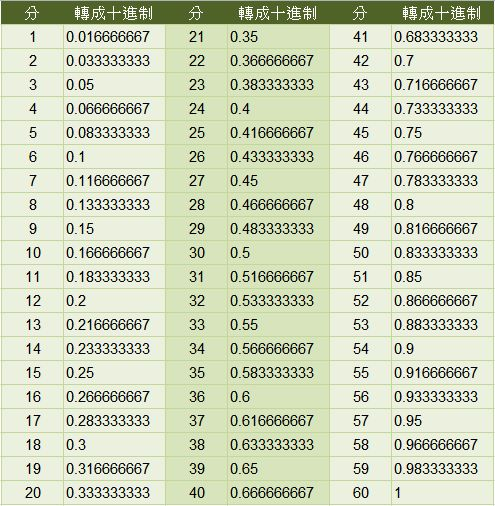• 把一个二进制转化为十进制数。 输入格式第一行一个正整数 n (1≤n≤30)，表示二进制数的长度。第二行一个二进制数。 输出格式输出一个整数，表示对应的十进制数。 样例输入 复制 5 ...
把一个二进制数转化为十进制数。 输入格式第一行一个正整数 n (1≤n≤30)，表示二进制数的长度。第二行一个二进制数。 输出格式输出一个整数，表示对应的十进制数。
                        样例输入                            复制
5
10101

样例输出                            复制
21

#include<stdio.h> #include<math.h> #include<string.h> int main() { int i,d,a,b,sum=0; char str; scanf("%d",&d); scanf("%s", str); for(i=0;i<d;i++) { a=str[i]-48; b=pow(2,d-1-i); sum+=a*b; } printf("%d\n",sum); return 0; }
这个48是ASCLL代码。 若有更好的建议的话，可以指教一下我这个小白。
展开全文• java二进制转化为十进制Here you will get program to convert binary to decimal in Java. 在这里，您将获得在Java中将二进制转换为十进制的程序。 There are mainly two ways to convert a binary number to ...java二进制转化为十进制
Here you will get program to convert binary to decimal in Java.
在这里，您将获得在Java中将二进制转换为十进制的程序。
There are mainly two ways to convert a binary number to decimal number in Java.
在Java中，主要有两种将二进制数转换为十进制数的方法。
1. By using parseInt() method of Integer class. 2. By using user defined logic.
1.通过使用Integer类的parseInt()方法。 2.通过使用用户定义的逻辑。
用Java将二进制转换为十进制的程序 (Program to Convert Binary to Decimal in Java)
By using Integer.parseInt()
通过使用Integer.parseInt()
Integer.parseInt() method takes two arguments. First argument is a string and second argument is the base or radix in which we have to convert the number. The output is the integer represented by the string argument in the specified radix. Below is the program for it.
Integer.parseInt()方法采用两个参数。 第一个参数是一个字符串，第二个参数是我们必须在其中转换数字的基数或基数。 输出是由指定基数中的字符串参数表示的整数。 下面是它的程序。
import java.util.Scanner;

class BinaryToDecimal
{
public static void main(String args[])
{
Scanner s=new Scanner(System.in);

System.out.println("Enter a binary number:");

String n=s.nextLine();

System.out.println(Integer.parseInt(n,2));
}
}
Without using Integer.parseInt()
不使用Integer.parseInt()
In this method we have to define our own logic for converting binary number to decimal. The approach that we will use here is mentioned in below example.
在这种方法中，我们必须定义自己的逻辑，以将二进制数转换为十进制。 下面的示例中提到了我们将在此处使用的方法。Image Source
图片来源
The program that implements above approach is mentioned below.
下面介绍实现上述方法的程序。
import java.util.Scanner;

class BinaryToDecimal
{
public static void main(String args[])
{
Scanner s=new Scanner(System.in);

System.out.println("Enter a binary number:");
int n=s.nextInt();

int decimal=0,p=0;

while(n!=0)
{
decimal+=((n%10)*Math.pow(2,p));
n=n/10;
p++;
}

System.out.println(decimal);
}
}
Output
输出量If you found anything missing or incorrect in above programs then please mention it by commenting below.
如果您在上述程序中发现任何丢失或不正确的内容，请在下面的评论中提及。

翻译自: https://www.thecrazyprogrammer.com/2015/11/program-to-convert-binary-to-decimal-in-java.html

java二进制转化为十进制

展开全文java 小程序 python 编程语言 spring
• c语言二进制转化为十进制Here you will get program to convert decimal to binary in C. 在这里，您将获得将C中的十进制转换二进制的程序。 We can convert a decimal number into binary by repeatedly ...c语言二进制转化为十进制
Here you will get program to convert decimal to binary in C.
在这里，您将获得将C中的十进制转换为二进制的程序。
We can convert a decimal number into binary by repeatedly dividing it by 2 and storing the remainder somewhere. Now display the remainders in reverse order.
我们可以通过将十进制数重复除以2并将剩余的数存储在某处来将其转换为二进制数。 现在以相反的顺序显示余数。
Also Read: Convert Binary to Decimal in C
另请参阅： 在C中将二进制转换为十进制
将十进制转换为C中的二进制 (Convert Decimal to Binary in C)
#include<stdio.h>

int main()
{
int d,n,i,j,a;
printf("Enter a number:");
scanf("%d",&n);

if(n==0)
printf("\nThe binary conversion of 0 is 0");
else
{
printf("\nThe binary conversion of %d is 1",n);

for(i=1;n!=1;++i)
{
d=n%2;
a[i]=d;
n=n/2;
}

for(j=i-1;j>0;--j)
printf("%d",a[j]);
}

return 0;
}
Output
输出量
Enter a number:10
输入数字：10
The binary conversion of 10 is 1010
10的二进制转换为1010

翻译自: https://www.thecrazyprogrammer.com/2013/02/c-program-to-convert-decimal-number-to.html

c语言二进制转化为十进制

展开全文python java c++ c语言 算法
• 实验目标：1.生成种群2.二进制转化为十进制3.交叉
• 适用于将二进制数转换为十进制，A为十进制，B为二进制。{A,B}每次左移一位，判断A的每四位是否＞4，若大于四则+3，否则保持不变；B多少位二进制数则左移多少次。最终A是B转换成十进制的数。代码32位二进制数转换...
• ## 二进制转化为十进制算法原理

千次阅读 多人点赞 2020-05-14 09:16:42
比如二进制1101，知换算成十进制就是：1*2（1-1）+0*2（2-1）+1*2（3-1）+1*2（4-1）=1+0+4+8=13。 扩展资道料： 1、二进制转换八进制： 把二进制的数从右往左，三位一组，不够补0 列：111=4+2+1=7 11001拆
从最低位（最右）算起，位上的数字乘以本位的权重，权重就百是2的第几位的位数减一次方。
比如第2位就是2的（2-1次）方，就是2；第8位就是2的（8-1）次方是128。把所有度的值加起来。
2（1-1）代表2的0次方，就是1；其他类推
比如二进制1101，知换算成十进制就是：1*2（1-1）+0*2（2-1）+1*2（3-1）+1*2（4-1）=1+0+4+8=13。扩展资道料：
1、二进制转换为八进制：
把二进制的数从右往左，三位一组，不够补0
列：111=4+2+1=7
11001拆分为 001和011，001=1，011=2+1=3。
那么11001转换为八进制就是31。
2、二进制转换为十六进制：
参照二进制转八内进制，但是它是从右往左，四位一组，不够补0
列子：1101101拆分为1101、0110
分别计算两个二进制的值，1101=8+4+0+1=13，十六进制中13为D
0110=4+2=6，那么二进制1101101转换为十六进制就是6D
展开全文• 二进制转化为十进制数 用字符型接收二进制的0、1然后判断是否‘1’ 功能实现 代码片. #include<iostream> using namespace std; double powe(double x, int n); int main() { int su = 0; char a; for ...c++
• } 结果 思路 用竖式来计算 注意接收的时候，除一个2就是在低位的，所以注意接收和输出的顺序，因为使用数组，所以第一个接收的其实是二进制的最低位，输出时应该倒序输出 注意 字符数组的初始化； 整型与字符型转化...
• Python将二进制变为十进制 直接上代码 参考自莫凡的遗传算法 def translate(pop): #... print("转化为十进制是："+str(a)) pop = np.random.randint(2, size=(1, 10)) print(pop) translate(pop) 首先使用numpypython
• 二进制转化为十进制 ①按权展开方法Java实现 /* * 按权展开法 */ public static double BinToTen1(String binary) { //查找改二进制是否存在小数点 int index = binary.indexOf('.'); //转化成的十进制 ...
• 二进制转化为十进制（除10取余法） 除10取余法中，对于二进制转化为10进制来讲要除于1010，就是把10转化二进制数，再用二进制数除于1010 例如：二进制11101除于1010，余数1001，转化为十进制9，这个是相当于...
• c语言编程-二进制转化为十进制 #include<stdio.h> static int a=0; double bintodec(char*str) { a=a*2+(*str-'0'); return 0; } int main() { char ch; while(ch=getchar(),ch!='\n') { char *p=&...
• 在Matlab中十进制转化为二进制矩阵，可以生成波形，比使用Matlab中自带的函数生成的char型结构更合理
• 二进制十进制，首先讲一下“权重”的概念 数字中某位的权重：2的（该位所在的位数（从右至左）-1）次方 比如：10 ...比如将10101转化为十进制 10101=1*2^4+0*2^3+1*2^2+0*2^1+1*2^0=21
• 八位二进制转三位十进制电路multisim源文件，十进制数字用数码管显示，multisim13及以上版本的软件可以正常打开仿真。
• 方便读者学习，本文小编给读者提供了用verilog将二进制码转换为十进制BCD码的程序设计方法，供读者参考。
• 本文给大家介绍的是一则使用C++实现读入二进制数并转换为十进制输出的代码，实现起来其实非常简单，C++本身就提供了二进制类库的，大家看代码吧，简单又实用。
• 本文主要讲了单片机二进制十进制程序代码，希望对你的学习有所帮助。
• b=input(“请输入一个二进制数:”) b_copy=b num=0 while b: num=num*2+eval(b) b=b[1:] print(“二进制数%s转化为十进制:%s”%(b_copy,num))
• 最近在做进制转化的FPGA实现，用到了FPGA的串口通信功能，首先将二进制数值（32定点数，末16位代表小数）由PC写入串口小助手，传给FPGA，再将转化后的结果由串口传到PC端显示，整体的过程比较简单，这部分以后再讲，...
• 使用C++语言实现十进制转换为二进制的运算方法，可正负互转C++
• 我们看到所转换的2进制数按底位到高位的顺序产生的，而通常的输出是从高位到低位的，恰好与计算过程相反，因此转换过程中每得到一位2进制数则进栈保存，转换完毕后依次出栈则正好是转换结果。 请实现其算法。java
• 分析：每个二进制转换相应的十进制方法：☺☺☺◆数学公式转化成算法◆☺☺☺ 看一下百度到的图片 上图第七位，其实是我们下面for循环体的第一位，所以才有了下面第i的2的次方：n.size()-i-1 -------------从...c++
• matlab开发-将二进制字符串转换为十进制值。它将二进制流转换为十进制值，每次8位，尽管您可以更改它。
•c++
• 十进制 表示法 103=1000102=100101=10两01 6= 6100 22 2101+210 358= 3102+5101+810 4699=4103+6102+9101+9100 二进制 表示法: 210=102429512282256 27=12826=64253 24=1623822=421=220=1 二进制转换成十进制 11=121...
• 已知一个只包含 0 和 1 的二进制数，长度不大于 10 ，将其转换为十进制并输出。 输入描述 输入一个二进制整数n，其长度大于0且不大于10 输出描述 输出转换后的十进制数， 占一行 样例输入 110 样例...C++
• /十进制二进制的三种方法/ package 数据结构; import java.util.Scanner;; public class 十转二进制 { public static void main(String[] args) { /*方法一：设置一个记位数的变量k; int k=1; //控制......